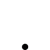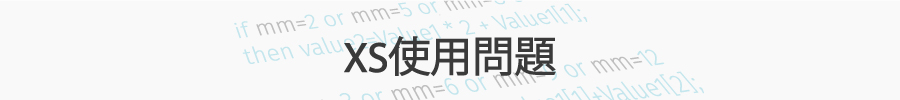關於特定時間出場問題

•   557
• 最後發表   河馬  2018 十二月 11

XQ小幫手 發文於   2018/11/07

Hi 河馬，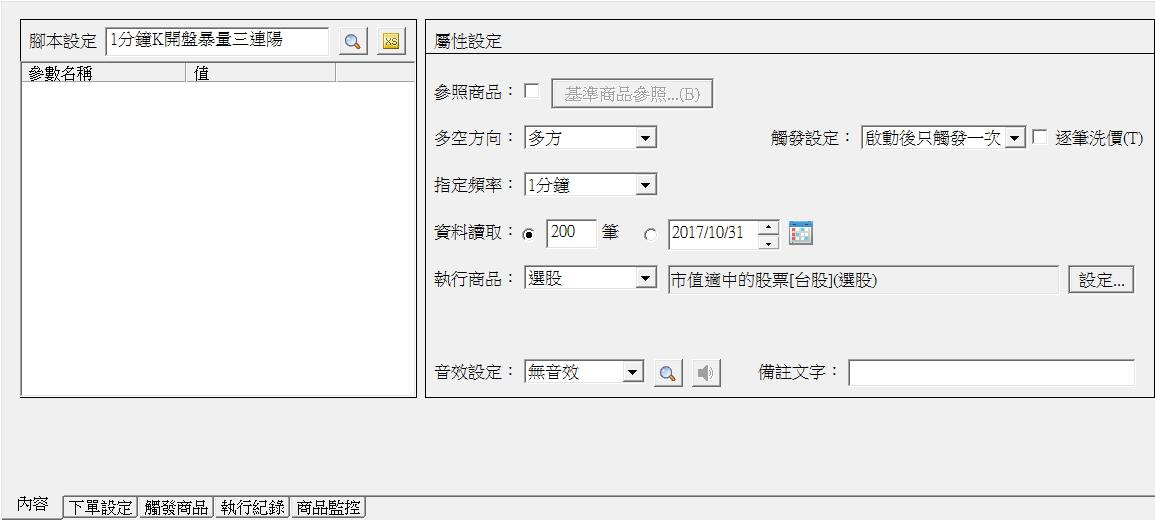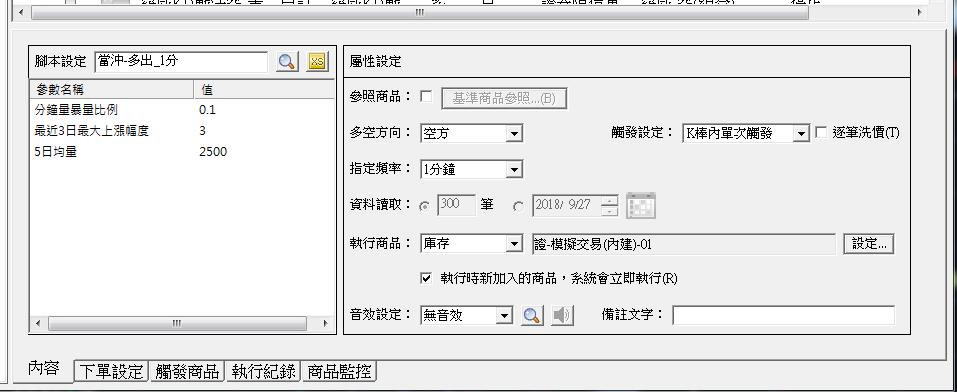XQ小幫手 發文於   2018/11/08

Hi 河馬，

//找了系統中的"開盤5分鐘三創新高"策略做為當沖基本策略進行修改測試，系統策略如下
//1.開高。
//2.五日均量大於1000張
//3.中小型股
//4.一分鐘線，開盤後扣除第一根之外的五根裡，至少有三根高點比前一根的高點高，而且收盤比前一根的收盤高
//5.前三天的漲幅不大
//6.這五根一分鐘線的成交量達到五日均量的一定比例
//7.大盤屬於多頭格局

input: volumeRatio(0.1, "分鐘量暴量比例");
input: changeRatio(3, "最近3日最大上漲幅度");
input: averageVolume(3000, "5日均量");

variable:KBarOfDay(0), BreakHigh(false), bycount(0);

KBarOfDay+=1;
if date<>date then begin
KBarOfDay=1;
BreakHigh = false;
bycount=0; //利用此變數控制每支個股一天只沖一次
end;

condition1 = KBarOfDay = 6;
//一分鐘線每天的第六根
condition2 = Countif(High > High and Close > Close ,5) >=3;
//近五根裡至少三根最高價比前一根高且收盤比前一根高

if KBarOfDay = 1
and close > getfield("close", "d") then BreakHigh = true;
//開高
value1 = average(GetField("Volume", "D"), 5);
//五日均量
condition3 = value1 > averageVolume;
//五日均量大於某張數
value2 = rateofchange(GetField("Close", "D"), 3);
condition4 = AbsValue(value2) < changeRatio;
//前三日漲幅小於一定標準
condition5 = summation(volume, 5) > value1 * volumeRatio;
//前五根一分鐘線成交量的合計大於五日均量某個比例
//GetSymbolField("TSE.TW","收盤價","D")>average(GetSymbolField("TSE.TW","收盤價","D"),10);
//大盤屬於多頭結構
settotalbar(300);
condition10=average(volume,5)>average(volume,25);
//5分均量大於25分均量
condition11=average(close,5)>average(close,25) and linearregslope(average(close,5),5)>0 and linearregslope(average(close,25),5)>0;
//5分均線須在25分均線上
condition12=average(getfield("close","5"),5)>average(getfield("close","5"),25) and linearregslope(average(getfield("close","5"),5),5)>0 and linearregslope(average(getfield("close","5"),25),5)>0;
//5五分均線須在25五分均線上

//進場
variable:byin(false),byprice(0);
if time<130000 and byin=false and bycount=0 and condition1 and condition2 and condition3
//and Condition4 and Condition5 and BreakHigh and condition6
and condition10 and condition11 and condition12 then
begin
byin=true;
byprice=close;
bycount=1;
end;

//退場
//移動停損
condition13=Countif(High < byprice,3) =3 and close < lowest(low,3);

if byin=true and condition13 then
begin
byin=false;
ret=1;
end;

//移動停利
if byin=true and lowest(low,6)>byprice then
begin
if close<lowest(low,3) then //跌破近3根低點退場
begin
byin=false;
ret=1;
end;

//固定退場，時間到13:15時，不論賺賠必須退場，不留至隔天
if byin=true and time>131500 then
begin
byin=false;
ret=1;
end;
end;

XQ小幫手 發文於   2018/11/09

Hi 河馬，

print(date,time,byin,condition1,condition2,condition3,condition10,condition11,condition12,KBarOfDay);

XQ小幫手 發文於   2018/11/12

Hi 河馬，

1. 進場腳本程式碼

2. 進場雷達設定（如 20181107 圖片範例）

1.進出場腳本是共用的，差別在ret=1指令的位置不同。

由於小幫手有說condition1難以成立，因此我提供一個修改過的版本，請小幫手以此版本測試

//找了系統中的"開盤5分鐘三創新高"策略做為當沖基本策略進行修改測試，系統策略如下
//1.開高。
//2.五日均量大於1000張
//3.中小型股
//4.一分鐘線，開盤後扣除第一根之外的五根裡，至少有三根高點比前一根的高點高，而且收盤比前一根的收盤高
//5.前三天的漲幅不大
//6.這五根一分鐘線的成交量達到五日均量的一定比例
//7.大盤屬於多頭格局

input: volumeRatio(0.1, "分鐘量暴量比例");
input: changeRatio(3, "最近3日最大上漲幅度");
input: averageVolume(3000, "5日均量");

variable:KBarOfDay(0), BreakHigh(false), bycount(0);

KBarOfDay+=1;
if date<>date then begin
KBarOfDay=1;
BreakHigh = false;
bycount=0; //利用此變數控制每支個股一天只沖一次
end;

condition1 = KBarOfDay >= 6;
//一分鐘線每天的第六根
condition2 = Countif(High > High and Close > Close ,5) >=3;
//近五根裡至少三根最高價比前一根高且收盤比前一根高

if KBarOfDay = 1
and close > getfield("close", "d") then BreakHigh = true;
//開高
value1 = average(GetField("Volume", "D"), 5);
//五日均量
condition3 = value1 > averageVolume;
//五日均量大於某張數
value2 = rateofchange(GetField("Close", "D"), 3);

settotalbar(300);

//進場
variable:byin(false),byprice(0);
if time<130000 and byin=false and bycount=0 and condition1 and condition2 and condition3
then
begin
byin=true;
ret=1;
byprice=close;
bycount=1;
end;

//退場
//移動停利
if byin=true and lowest(low,6)>byprice then
begin
if close<lowest(low,3) then //跌破近3根低點退場
begin
byin=false;
end;
end;

//固定退場，時間到13:15時，不論賺賠必須退場，不留至隔天
if byin=true and time>131500 then
begin
byin=false;
end;

//找了系統中的"開盤5分鐘三創新高"策略做為當沖基本策略進行修改測試，系統策略如下
//1.開高。
//2.五日均量大於1000張
//3.中小型股
//4.一分鐘線，開盤後扣除第一根之外的五根裡，至少有三根高點比前一根的高點高，而且收盤比前一根的收盤高
//5.前三天的漲幅不大
//6.這五根一分鐘線的成交量達到五日均量的一定比例
//7.大盤屬於多頭格局

input: volumeRatio(0.1, "分鐘量暴量比例");
input: changeRatio(3, "最近3日最大上漲幅度");
input: averageVolume(3000, "5日均量");

variable:KBarOfDay(0), BreakHigh(false), bycount(0);

KBarOfDay+=1;
if date<>date then begin
KBarOfDay=1;
BreakHigh = false;
bycount=0; //利用此變數控制每支個股一天只沖一次
end;

condition1 = KBarOfDay >= 6;
//一分鐘線每天的第六根
condition2 = Countif(High > High and Close > Close ,5) >=3;
//近五根裡至少三根最高價比前一根高且收盤比前一根高

if KBarOfDay = 1
and close > getfield("close", "d") then BreakHigh = true;
//開高
value1 = average(GetField("Volume", "D"), 5);
//五日均量
condition3 = value1 > averageVolume;
//五日均量大於某張數
value2 = rateofchange(GetField("Close", "D"), 3);

settotalbar(300);

//進場
variable:byin(false),byprice(0);
if time<130000 and byin=false and bycount=0 and condition1 and condition2 and condition3
then
begin
byin=true;
byprice=close;
bycount=1;
end;

//退場
//移動停利
if byin=true and lowest(low,6)>byprice then
begin
if close<lowest(low,3) then //跌破近3根低點退場
begin
byin=false;
ret=1;
end;
end;

//固定退場，時間到13:15時，不論賺賠必須退場，不留至隔天
if byin=true and time>131500 then
begin
byin=false;
ret=1;
end;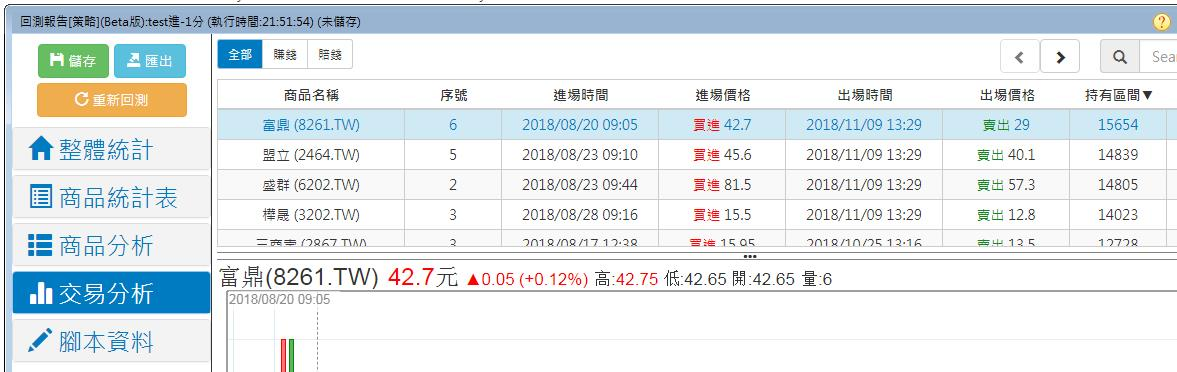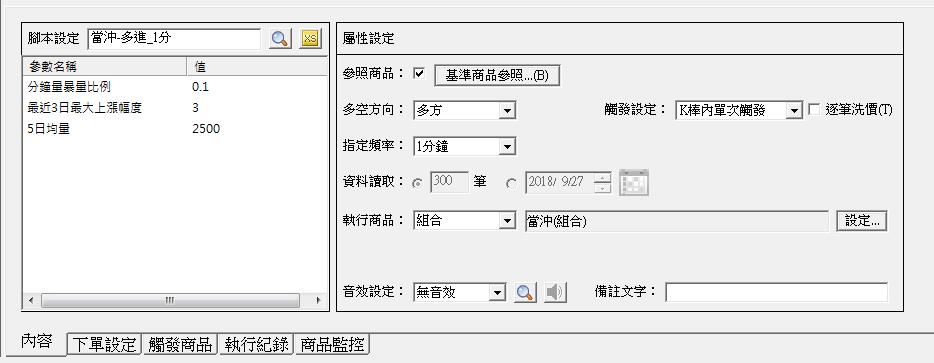XQ小幫手 發文於   2018/11/13

Hi 河馬，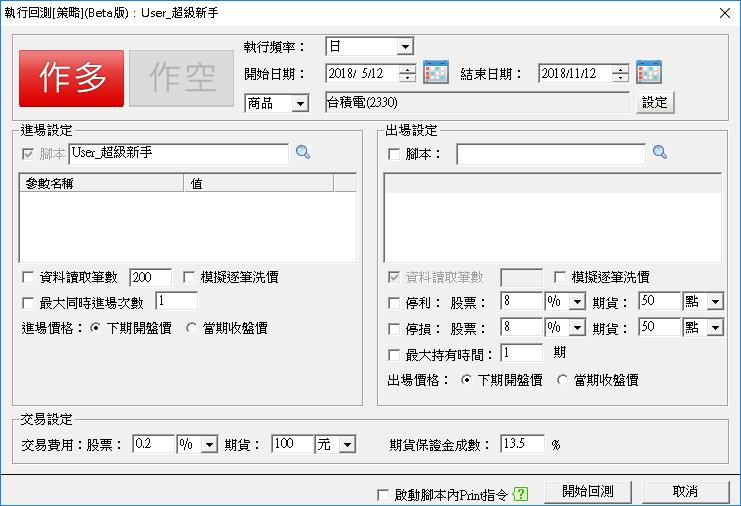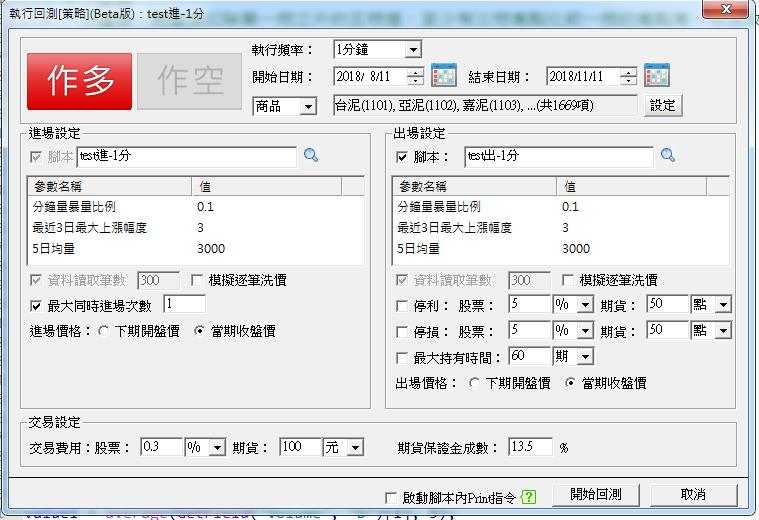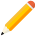發表新主題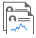我的發文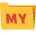我的收藏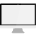分類